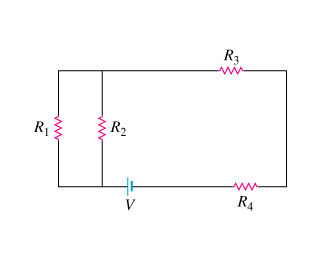# Problem: Consider the network of four resistors shown in the diagram, where R1 = 2.00? , R2 = 5.00? , R3 = 1.00? , and R4 = 7.00? . The resistors are connected to a constant voltage of magnitude V. (Figure 1)A. Find the equivalent resistance RA of the resistor network.

###### FREE Expert Solution

Equivalent resistance for 2 resistors in parallel:

$\overline{){{\mathbf{R}}}_{{\mathbf{eq}}}{\mathbf{=}}\frac{{\mathbf{R}}_{\mathbf{1}}{\mathbf{R}}_{\mathbf{2}}}{{\mathbf{R}}_{\mathbf{1}}\mathbf{+}{\mathbf{R}}_{\mathbf{2}}}}$

Equivalent resistance for resistors in series:

$\overline{){{\mathbf{R}}}_{{\mathbf{eq}}}{\mathbf{=}}{{\mathbf{R}}}_{{\mathbf{1}}}{\mathbf{+}}{{\mathbf{R}}}_{{\mathbf{2}}}{\mathbf{+}}{\mathbf{.}}{\mathbf{.}}{\mathbf{.}}{\mathbf{+}}{{\mathbf{R}}}_{{\mathbf{n}}}}$

R1 and R2 are in parallel.

90% (37 ratings)###### Problem DetailsConsider the network of four resistors shown in the diagram, where R1 = 2.00? , R2 = 5.00? , R3 = 1.00? , and R4 = 7.00? . The resistors are connected to a constant voltage of magnitude V. (Figure 1)

A. Find the equivalent resistance RA of the resistor network.